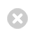Close

MPHY0021: Research Software Engineering With Python

Home## Plotting with Matplotlib¶

Plotting data is very common and useful in scientific work. Python does not include any plotting functionality in the language itself, but there are various frameworks available for producing plots and visualisations.

In this section, we will look at Matplotlib, one of those frameworks. As the name indicates, it was conceived to provide an interface similar to the MATLAB programming language, but no knowledge of MATLAB is required!

### Importing Matplotlib¶

We import the pyplot object from Matplotlib, which provides us with an interface for making figures. We usually abbreviate it.

In :
from matplotlib import pyplot as plt


### Notebook magics¶

When we write:

In :
%matplotlib inline


We tell the Jupyter notebook to show figures we generate alongside the code that created it, rather than in a separate window. Lines beginning with a single percent are not python code: they control how the notebook deals with python code.

Lines beginning with two percents are "cell magics", that tell Jupyter notebook how to interpret the particular cell; we've seen %%writefile, for example.

### A basic plot¶

When we write:

In :
from math import sin, cos, pi
my_fig = plt.plot([sin(pi * x / 100.0) for x in range(100)])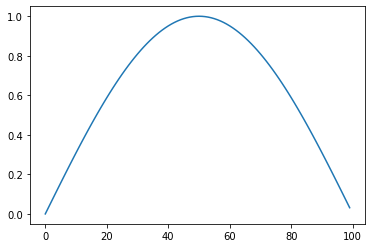The plot command returns a figure, just like the return value of any function. The notebook then displays this.

To add a title, axis labels etc, we need to get that figure object, and manipulate it. For convenience, matplotlib allows us to do this just by issuing commands to change the "current figure":

In :
plt.plot([sin(pi * x / 100.0) for x in range(100)])
plt.title("Hello")

Out:
Text(0.5, 1.0, 'Hello')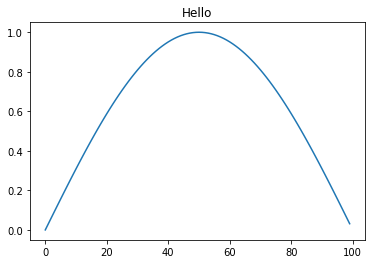But this requires us to keep all our commands together in a single cell, and makes use of a "global" single "current plot", which, while convenient for quick exploratory sketches, is a bit cumbersome. To produce from our notebook proper plots to use in papers, the library defines some types we can use to treat individual figures as variables, and manipulate these.

### Figures and Axes¶

We often want multiple graphs in a single figure (e.g. for figures which display a matrix of graphs of different variables for comparison).

So Matplotlib divides a figure object up into axes: each pair of axes is one 'subplot'. To make a boring figure with just one pair of axes, however, we can just ask for a default new figure, with brand new axes. The relevant function returns a (figure, axis) pair, which we can deal out with parallel assignment (unpacking).

In :
sine_graph, sine_graph_axes = plt.subplots()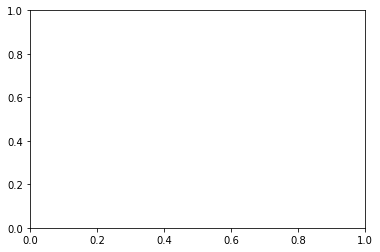Once we have some axes, we can plot a graph on them:

In :
sine_graph_axes.plot([sin(pi * x / 100.0) for x in range(100)], label='sin(x)')

Out:
[<matplotlib.lines.Line2D at 0x7f1772f3dd90>]

We can add a title to a pair of axes:

In :
sine_graph_axes.set_title("My graph")

Out:
Text(0.5, 1.0, 'My graph')
In :
sine_graph_axes.set_ylabel("f(x)")

Out:
Text(3.200000000000003, 0.5, 'f(x)')
In :
sine_graph_axes.set_xlabel("100 x")

Out:
Text(0.5, 3.1999999999999993, '100 x')

Now we need to actually display the figure. As always with the notebook, if we make a variable be returned by the last line of a code cell, it gets displayed:

In :
sine_graph

Out: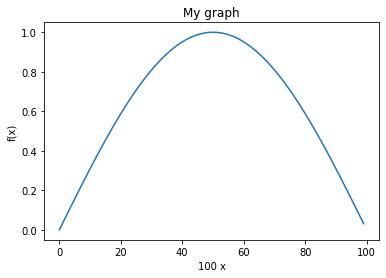In :
sine_graph_axes.plot([cos(pi * x / 100.0) for x in range(100)], label='cos(x)')

Out:
[<matplotlib.lines.Line2D at 0x7f1772f3df40>]
In :
sine_graph

Out: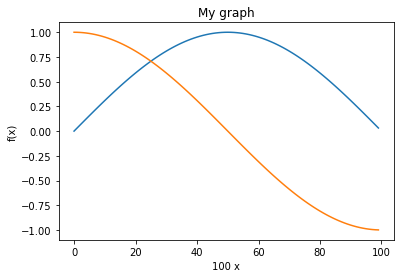A legend will help us distinguish the curves:

In :
sine_graph_axes.legend()

Out:
<matplotlib.legend.Legend at 0x7f1772f64ca0>
In :
sine_graph

Out: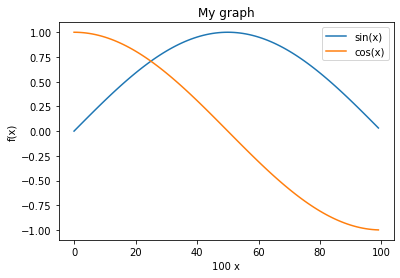### Saving figures¶

We must be able to save figures to disk, in order to use them in papers. This is really easy:

In :
sine_graph.savefig('my_graph.png')


In order to be able to check that it worked, we need to know how to display an arbitrary image in the notebook.

The programmatic way is like this:

In :
from IPython.display import Image # Get the notebook's own library for manipulating itself.
Image(filename='my_graph.png')

Out: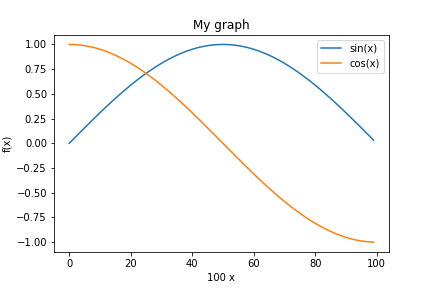### Subplots¶

We might have wanted the $\sin$ and $\cos$ graphs on separate axes:

In :
double_graph = plt.figure()

<Figure size 432x288 with 0 Axes>
In :
sin_axes = double_graph.add_subplot(2, 1, 1) # 2 rows, 1 column, 1st subplot

In :
cos_axes = double_graph.add_subplot(2, 1, 2) # 2 rows, 1 column, 2nd subplot

In :
double_graph

Out: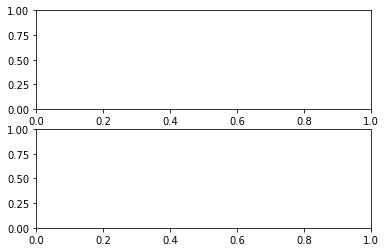In :
sin_axes.plot([sin(pi * x / 100.0) for x in range(100)])

Out:
[<matplotlib.lines.Line2D at 0x7f1772e2abb0>]
In :
sin_axes.set_ylabel("sin(x)")

Out:
Text(3.200000000000003, 0.5, 'sin(x)')
In :
cos_axes.plot([cos(pi * x / 100.0) for x in range(100)])

Out:
[<matplotlib.lines.Line2D at 0x7f1772e2a4f0>]
In :
cos_axes.set_ylabel("cos(x)")

Out:
Text(3.200000000000003, 0.5, 'cos(x)')
In :
cos_axes.set_xlabel("100 x")

Out:
Text(0.5, 3.200000000000003, '100 x')
In :
double_graph

Out: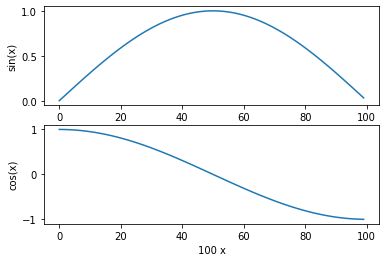### Versus plots¶

When we specify a single list to plot, the x-values are just the array index number. We usually want to plot something more meaningful:

In :
double_graph = plt.figure()
cos_axes.set_ylabel("cos(x)")
sin_axes.set_ylabel("sin(x)")
cos_axes.set_xlabel("x")

Out:
Text(0.5, 0, 'x')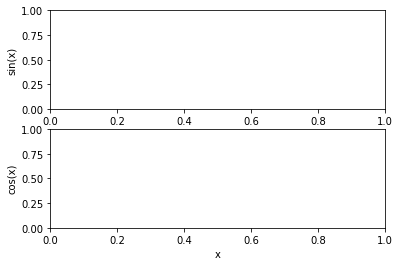In :
sin_axes.plot([x / 100.0 for x in range(100)], [sin(pi * x / 100.0) for x in range(100)])
cos_axes.plot([x / 100.0 for x in range(100)], [cos(pi * x / 100.0) for x in range(100)])

Out:
[<matplotlib.lines.Line2D at 0x7f1772d5f970>]
In :
double_graph

Out: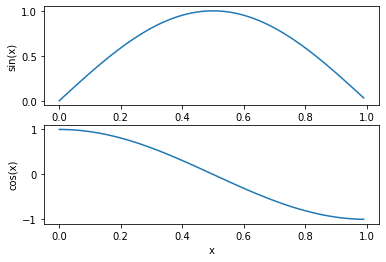### Learning More¶

There's so much more to learn about matplotlib: pie charts, bar charts, heat maps, 3-d plotting, animated plots, and so on. You can learn all this via the Matplotlib Website. You should try to get comfortable with all this, so please use some time in class, or at home, to work your way through a bunch of the examples.